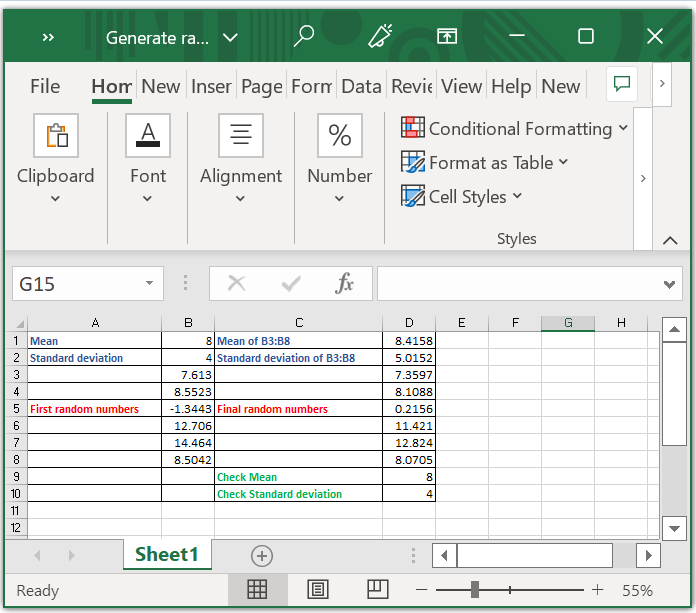# Generate random numbers by giving certain mean and standard deviation in Excel

There may be times when you need to generate a series of random numbers based on specific mean and standard deviation for some scenarios.

## Generate random numbers by given Mean and Standard Deviation

To solve this, you need to follow the below step-by-step process.

Step 1

To begin with, you need to enter your needed mean and standard deviation into two empty cells, in this case, I have selected A1 and A2 as shown in the below screenshot.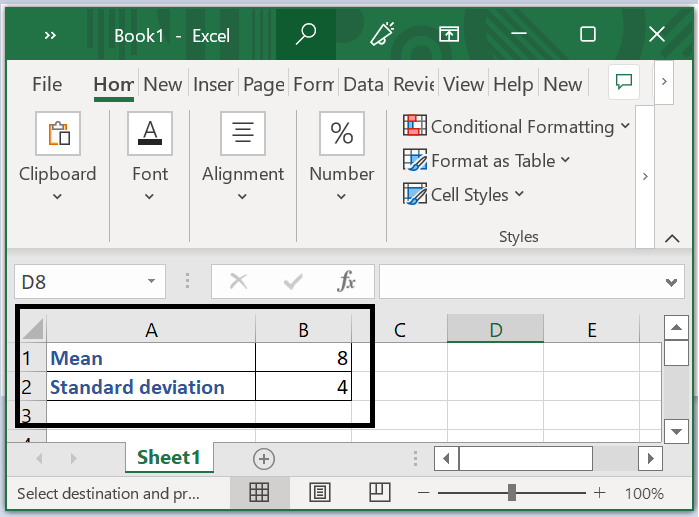Step 2

Afterwards, in cell B3, type this formula =NORMINV(RAND(),$B$1,$B$2) and drag the handle to the desired range in order to fill the cell as shown in below screenshot.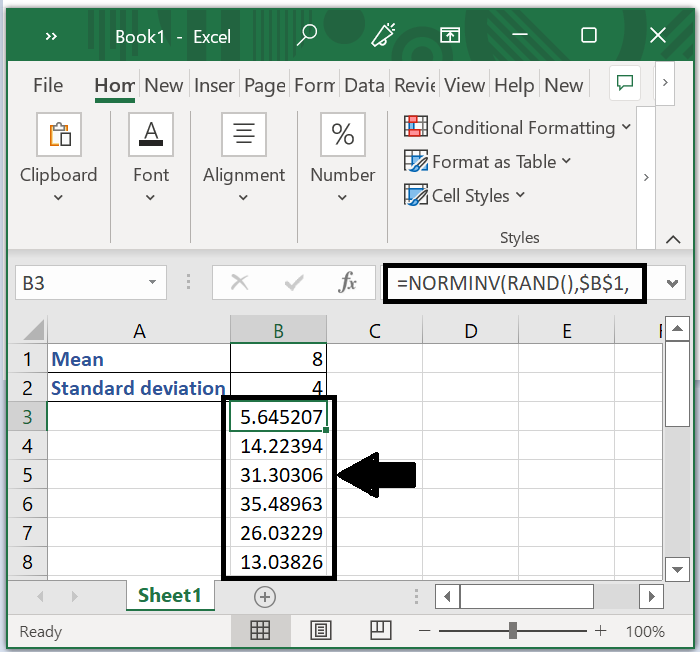In the formula =NORMINV(RAND(),$B$1,$B$2), $B1 indicates the mean,$B$2 indicates the standard deviation. Step 3 In the cell D1 and D2 you have to calculate the mean and standard deviation of the random number you have entered in step 2. In the cell D1, calculate the mean by entering the formula =AVERAGE(B3:B8), press Enter key. In D2, calculate the standard deviation for that enter the formula =STDEV.P(B3:B8) and press Enter key as shown in the below screenshot.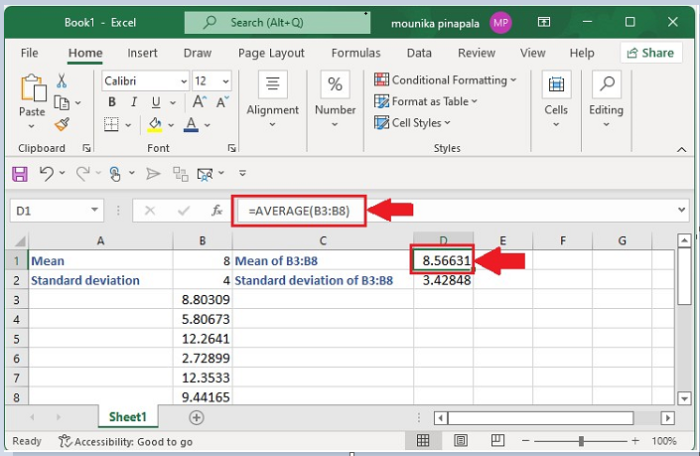Below screenshot with the formula to calculate standard deviation of B3:B8.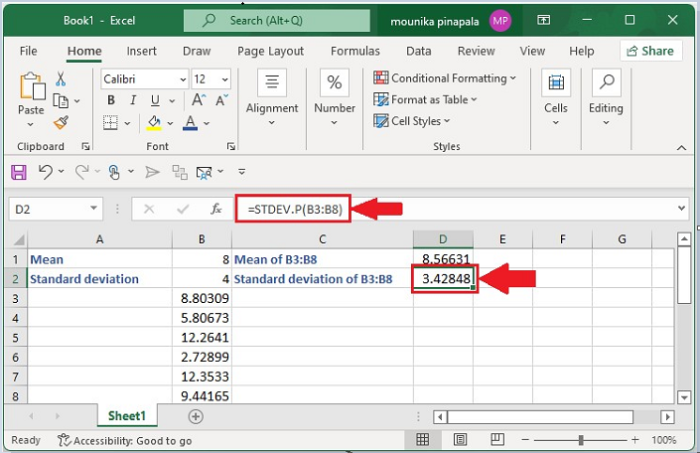Here B3:B8 is the range you selected the numbers in step 2. Step 4 You can generate the real random numbers that you want. In the cell D3, enter the formula =$B$1+(B3-$D$1)*$B$2/$D\$2 and drag till B8 as in the above we have considered till B8. Below is the screenshot.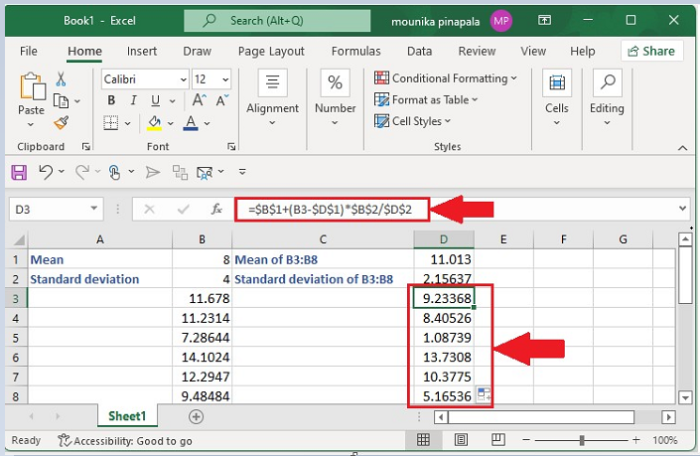Here B1 indicates the mean you need B2 indicates the standard deviation you need. B3 indicates the first cell of your first random numbers, D1 indicated the mean of the first random number. D2 is the standard deviation of first random numbers.

Step 5

You can check the mean and standard deviation of the final random number series. In the cell D9, enter the formula =AVERAGE(D3:D8) and press enter key and then in the cell D10 enter the formula =STDEV.P(D3:D8) and press enter key as shown below −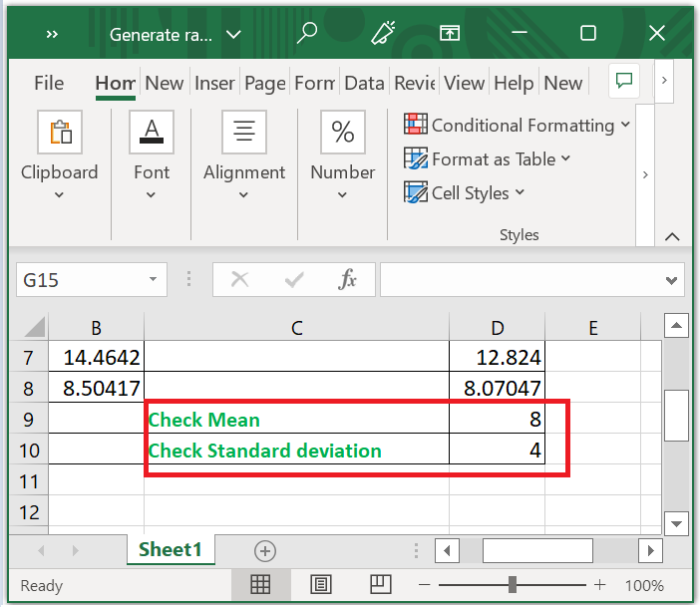Here D3 to D8 is the range of the last random number series.

Left side of the data in the Excel sheet is First random numbers and right side of the data is Final random numbers as shown below.